# GMAT Math : DSQ: Understanding measurement

## Example Questions

← Previous 1

### Example Question #1 : Dsq: Understanding Measurement

A really backward country does not use inches, feet, yards, or meters to measure distance, it uses units called wumps and zumps. These units can also be used to form units of area and volume (i.e. square wumps, cubic zumps).

How many wumps are in one zump?

Statement 1: There are 361 square wumps in one square zump.

Statement 2: There are 6,859 cubic wumps in one cubic zump.

BOTH statements TOGETHER are insufficient to answer the question.

EITHER statement ALONE is sufficient to answer the question.

Statement 2 ALONE is sufficient to answer the question, but Statement 1 ALONE is NOT sufficient to answer the question.

BOTH statements TOGETHER are sufficient to answer the question, but NEITHER statement ALONE is sufficient to answer the question.

Statement 1 ALONE is sufficient to answer the question, but Statement 2 ALONE is NOT sufficient to answer the question.

EITHER statement ALONE is sufficient to answer the question.

Explanation:

If there arewumps in a zump, then there are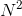square wump in a square zump, and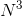cubic wump in a cubic zump. Therefore, given the first statement, you can take the square root of the conversion factor to get the number of wumps in a zump; given the second, you can take the cube root of that conversion factor to the same end. In both cases you get 19.

### Example Question #2 : Dsq: Understanding Measurement

You are given a metal cube whose volume can be given by a whole number of cubic meters. What is its volume?

Statement 1: Each edge of the cube has length between 160 and 180 centimeters, inclusive.

Statement 2: The surface area of the cube is between 1,350 square decimeters and 1,944 square decimeters, inclusive.

EITHER statement ALONE is sufficient to answer the question.

BOTH statements TOGETHER are sufficient to answer the question, but NEITHER statement ALONE is sufficient to answer the question.

BOTH statements TOGETHER are insufficient to answer the question.

Statement 1 ALONE is sufficient to answer the question, but Statement 2 ALONE is NOT sufficient to answer the question.

Statement 2 ALONE is sufficient to answer the question, but Statement 1 ALONE is NOT sufficient to answer the question.

Statement 1 ALONE is sufficient to answer the question, but Statement 2 ALONE is NOT sufficient to answer the question.

Explanation:

Assume Statement 1 alone. Each edge of the cube has length between 160 centimeters and 180 centimeters; since one meter is equal to 100 centimeters, the length of one edge falls between 1.6 meters and 1.8 meters. Its volume therefore falls between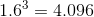cubic meters

and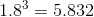cubic meters.

The only whole number of meters that falls between these boundaries is 5 meters, so the question is answered.

Assume Statement 2 alone. The minimum surface area of the cube is 1,350 square decimeters, so, to find the minumum length of an edge, use the surface area formula, setting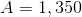: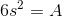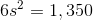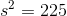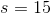decimeters; divide by 10 (decimeters per meter) to convert to 1.5 meters.

Similarly, to get the maximum edge length, set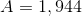: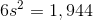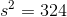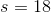decimeters; divide by 10 to get 1.8 meters.

The volume of the cube therefore falls between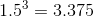cubic meters

andcubic meters.

This leaves us with two possible whole number answers, 4 and 5, so the question is not answered.

### Example Question #3 : Dsq: Understanding Measurement

You are given a square metal sheet whose area can be given by a whole number of square feet. What is the length of each side?

Statement 1: The length of each side is between 24 and 32 inches, inclusive.

Statement 2: The area of the sheet is no greater than one half of a square yard.

BOTH statements TOGETHER are sufficient to answer the question, but NEITHER statement ALONE is sufficient to answer the question.

Statement 2 ALONE is sufficient to answer the question, but Statement 1 ALONE is NOT sufficient to answer the question.

Statement 1 ALONE is sufficient to answer the question, but Statement 2 ALONE is NOT sufficient to answer the question.

BOTH statements TOGETHER are insufficient to answer the question.

EITHER statement ALONE is sufficient to answer the question.

BOTH statements TOGETHER are sufficient to answer the question, but NEITHER statement ALONE is sufficient to answer the question.

Explanation:

Assume Statement 1 alone.

24 inches is equal to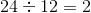feet, and 32 inches is equal to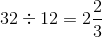feet. If, as given in Statement 1, the length of each side of a square falls between these measures, the range of the area is as follows: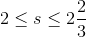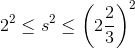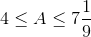This gives us four possible whole numbers of square feet for the area - 4, 5, 6, and 7.

Assume Statement 2 alone. One yard comprises three feet, so one square yard comprises nine square feet. One half of a square yard is equal to half of nine, or four and a half, square feet. This allows us to narrow down the area to four whole numbers of square feet - 1, 2, 3, or 4.

Neither statement alone is sufficient, but both are - since 4 square feet is the only possibility that satisfies both statements, it can be found to be the answer to the question if both are assumed.

### Example Question #4 : Dsq: Understanding Measurement

You are given a metal cube whose volume can be given as a whole number of cubic yards. What is its volume?

Statement 1: Each edge has length between 6 feet and 7 feet, inclusive.

Statement 2: The area of each face of the cube is between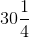square feet and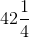square feet, inclusive.

EITHER statement ALONE is sufficient to answer the question.

BOTH statements TOGETHER are sufficient to answer the question, but NEITHER statement ALONE is sufficient to answer the question.

Statement 2 ALONE is sufficient to answer the question, but Statement 1 ALONE is NOT sufficient to answer the question.

BOTH statements TOGETHER are insufficient to answer the question.

Statement 1 ALONE is sufficient to answer the question, but Statement 2 ALONE is NOT sufficient to answer the question.

BOTH statements TOGETHER are insufficient to answer the question.

Explanation:

Assume both statements are true. If we letbe the length of one side in yards, then from Statement 1, we can divide maximum and minimum lengths by 3 to obtain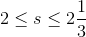From Statement 2 alone, We can take the square root of the maximum and minmum areas to obtain the maximum and minimum areas in feet, then divide by three to convert these to yards: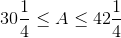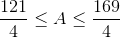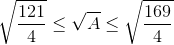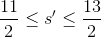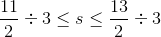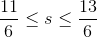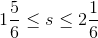(NOTE: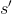is being used for sidelength in feet).

From the two statements together, we obtain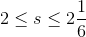The maximum and minimum values of the volume are the cubes of those of the sidelengths: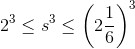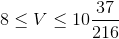The volume must be a whole number of cubic yards, so it must be 8, 9, or 10 - but without additional information, we cannot narrow it down further.

### Example Question #5 : Dsq: Understanding Measurement

You are given a square metal sheet whose area can be given by a whole number of square feet. What is the length of each side?

Statement 1: The area of the sheet is between 3 square yards and 4 square yards inclusive.

Statement 2: Each side of the sheet measures between 60 and 72 inches inclusive.

Statement 2 ALONE is sufficient to answer the question, but Statement 1 ALONE is NOT sufficient to answer the question.

Statement 1 ALONE is sufficient to answer the question, but Statement 2 ALONE is NOT sufficient to answer the question.

BOTH statements TOGETHER are sufficient to answer the question, but NEITHER statement ALONE is sufficient to answer the question.

EITHER statement ALONE is sufficient to answer the question.

BOTH statements TOGETHER are insufficient to answer the question.

BOTH statements TOGETHER are insufficient to answer the question.

Explanation:

Examine Statement 1. One yard comprises three feet, so one square yard comprises nine square feet. Three square yards is equal to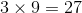square feet, and four square yards is equal to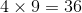square feet. Therefore, the area in feet is one of the whole numbers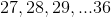.

Examine Statement 2. 60 inches is equal to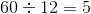feet, and 72 inches is equal to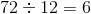square feet. The range of areas of the square sheet can be found as follows: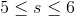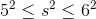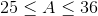Therefore, the area in feet is one of the whole numbers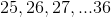.

Therefore, the two statements together yield ten possible answers - the whole numbers from 27 to 36.

### Example Question #6 : Dsq: Understanding Measurement

The length of a metal rod can be given by a whole number of inches. How long is the rod?

Statement 1: If four inches are removed from each end, then the length can be given in a whole number of feet.

Statement 2: If twenty inches are removed from one end, then the length can be given in a whole number of yards.

Statement 1 ALONE is sufficient to answer the question, but Statement 2 ALONE is NOT sufficient to answer the question.

BOTH statements TOGETHER are insufficient to answer the question.

BOTH statements TOGETHER are sufficient to answer the question, but NEITHER statement ALONE is sufficient to answer the question.

EITHER statement ALONE is sufficient to answer the question.

Statement 2 ALONE is sufficient to answer the question, but Statement 1 ALONE is NOT sufficient to answer the question.

BOTH statements TOGETHER are insufficient to answer the question.

Explanation:

Assume both statements to be true. Examine these two scenarios.

Case 1: The metal rod is 56 inches long.

If four inches are removed from each end - that is, if eight inches total are removed - the rod will have length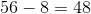inches, which is equal to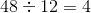feet.

If twenty inches are removed, the rod will have length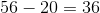inches, which is equal to one yard.

Case 2: The metal rod is 92 inches long.

If four inches are removed from each end - that is, if eight inches total are removed - the rod will have length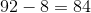inches, which is equal to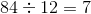feet.

If twenty inches are removed, the rod will have length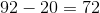inches, which is equal to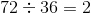yards.

Both lengths satisfy the conditions given in both statements, so, together, the statements provide insufficient information.

### Example Question #7 : Dsq: Understanding Measurement

You are given a square metal sheet whose area can be given by a whole number of square meters. What is the length of each side?

Statement 1: The length of each side of the sheet is between 7 and 8 feet, inclusive.

Statement 2: The area of the sheet is between 40 and 60 square feet, inclusive.

NOTE: 1 meter = about 3.3 feet.

EITHER statement ALONE is sufficient to answer the question.

BOTH statements TOGETHER are sufficient to answer the question, but NEITHER statement ALONE is sufficient to answer the question.

BOTH statements TOGETHER are insufficient to answer the question.

Statement 1 ALONE is sufficient to answer the question, but Statement 2 ALONE is NOT sufficient to answer the question.

Statement 2 ALONE is sufficient to answer the question, but Statement 1 ALONE is NOT sufficient to answer the question.

Statement 1 ALONE is sufficient to answer the question, but Statement 2 ALONE is NOT sufficient to answer the question.

Explanation:

Assume Statement 1 alone. Since one meter is equal to about 3.3 feet, we can divide the minimum and maximum lengths in feet by 3.3 to get these lengths in meters:

Minimum length: 7 feet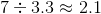meters

Maximum length: 8 feet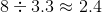meters

The minimum and maximum areas of the sheet in square meters are the squares of these: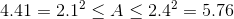Since the area of the sheet must be a whole number of square meters, the only possble area is 5 square meters.

Assume Statement 2 alone. Since one meter is equal to about 3.3 feet, we square this to get the conversion factor from square meters to square feet:

One square meter is equal to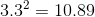square feet.

Divide the minimum and maximum areas in square feet by 10.89 to convert them to square meters:

Minimum area: 40 square feet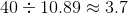square meters:

Maximum area: 60 square feet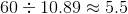square meters:

The area in square meters is a whole number between 3.7 and 5.5, so the area in square meters is either 4 or 5 . Without additional information, however, we cannot narrow it down further.

### Example Question #8 : Dsq: Understanding Measurement

The weight of the coffee in a can be given by a whole number of ounces. How many ounces does it weigh?

Statement 1: If eight ounces of coffee are removed, what is left over can be expressed as a whole number of pounds.

Statement 2: There are between 50 and 60 ounces of coffee in the can.

Statement 1 ALONE is sufficient to answer the question, but Statement 2 ALONE is NOT sufficient to answer the question.

BOTH statements TOGETHER are insufficient to answer the question.

BOTH statements TOGETHER are sufficient to answer the question, but NEITHER statement ALONE is sufficient to answer the question.

Statement 2 ALONE is sufficient to answer the question, but Statement 1 ALONE is NOT sufficient to answer the question.

EITHER statement ALONE is sufficient to answer the question.

BOTH statements TOGETHER are sufficient to answer the question, but NEITHER statement ALONE is sufficient to answer the question.

Explanation:

From Statement 1 alone, it can be determined that there are eight ounces more that a whole number of pounds; equivalently, the number of ounces in can is 8 greater than a multiple of 16, such as 24, 40, 66, etc. However, we cannot narrow it down further.

From Statement 2 alone, we can narrow the possibilities down to eleven: 50, 51, up to 60 ounces. We cannot narrow it down further.

Assume both statements to be true. We can start with 8 and keep adding 16 to find a number that 8 greater than a multiple of 16 and that is between 50 and 60: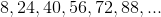The only possible weight of the coffee is 56 ounces.

### Example Question #9 : Dsq: Understanding Measurement

You are given a square metal sheet whose area can be given by a whole number of square yards. What is the length of each side?

Statement 1: Each side has length between 4 and 6 feet inclusive.

Statement 2: Each side has length between 44 and 54 inches inclusive.

Statement 1 ALONE is sufficient to answer the question, but Statement 2 ALONE is NOT sufficient to answer the question.

EITHER statement ALONE is sufficient to answer the question.

BOTH statements TOGETHER are sufficient to answer the question, but NEITHER statement ALONE is sufficient to answer the question.

BOTH statements TOGETHER are insufficient to answer the question.

Statement 2 ALONE is sufficient to answer the question, but Statement 1 ALONE is NOT sufficient to answer the question.

Statement 2 ALONE is sufficient to answer the question, but Statement 1 ALONE is NOT sufficient to answer the question.

Explanation:

Assume Statement 1 alone. Since the length of one side is at minimum 4 feet, or equivalently,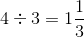yards, its area is at least the square of this, or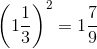square yards. Since the length of one side is at most 6 feet, or, equivalently,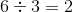yards, its area is at most the square of this, or 4 square yards. Since the area must be equal to a whole number of yards, we can only narrow the area down to 2, 3, or 4 square yards.

Assume Statement 2 alone. Since the length of one side is at minimum 44 inches, or equivalently,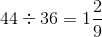yards, its area is at least the square of this, or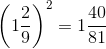square yards. Since the length of one side is at most 54 inches, or, equivalently,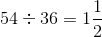yards, its area is at most the square of this, or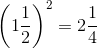square yards. Since the area must be equal to a whole number of yards, the only possible area for the sheet is 2 square yards.

### Example Question #1 : Measurement Problems

The weight of a bag of oranges can be given by a whole number of ounces. What is its weight?

Statement 1: The bag of oranges weighs between 110 ounces and 130 ounces inclusive.

Statement 2: The weight of the bag of oranges can be given as a whole number of pounds.

BOTH statements TOGETHER are sufficient to answer the question, but NEITHER statement ALONE is sufficient to answer the question.

EITHER statement ALONE is sufficient to answer the question.

Statement 2 ALONE is sufficient to answer the question, but Statement 1 ALONE is NOT sufficient to answer the question.

Statement 1 ALONE is sufficient to answer the question, but Statement 2 ALONE is NOT sufficient to answer the question.

BOTH statements TOGETHER are insufficient to answer the question.

BOTH statements TOGETHER are insufficient to answer the question.

Explanation:

Assume both statements are true. Since, as known from Statement 2, the weight of the bag of oranges is given by a whole number of pounds, then the weight in ounces must be a multiple of 16. From Statement 1, this number is between 110 and 130 inclusive. However, there are two multiples of 16 - 112 and 128 - that fall in this range. Therefore, the question cannot be answered for certain.

← Previous 1

Tired of practice problems?

Try live online GMAT Math prep today.